## The resonance tube used in this experiment produced only one resonance tone. What length of tube would be required to produce a second tone

Question

The resonance tube used in this experiment produced only one resonance tone. What length of tube would be required to produce a second tone under the same experimental conditions? Explain your answer.

in progress 0
5 months 2021-08-15T07:08:39+00:00 1 Answers 10 views 0

the length that would produce a sound tone under the same experimental contditions must be increased by  Δl =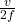Explanation:

Recall

V = f ×λ

where λ is ⁴/₃l₂ for second resonance

f =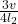l₂ =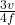where λ is 4l₁ for 1st resonance

f =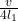l₁ =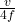∴ Δl = l₂ – l₁ =⁻Δl=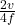Δl =Therefore, the length should increase by